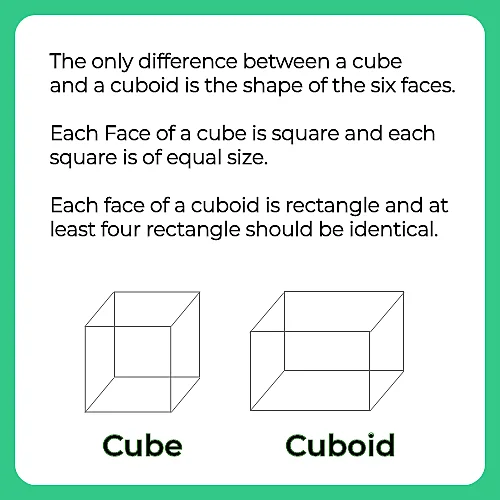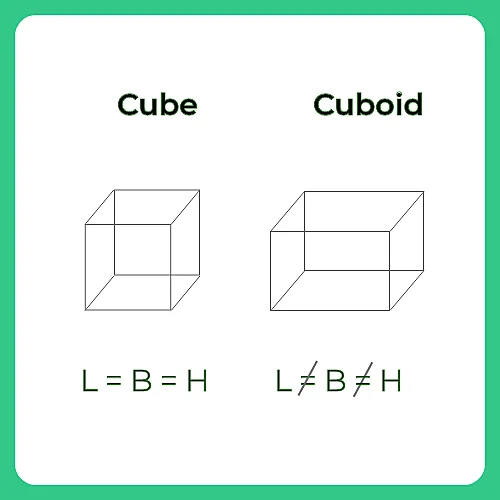# How To Solve Cube And Cuboid Quickly

## Surface Area of Cuboid:### Surface Area of Cube:

A cube is a 3d representation of a square and has all equal sides. The length, breadth, and height in a cube are the same and are termed as sides (s). If s is each edge of the cube then, the surface area of a cube:

• 2 [(s × s) + (s × s) + (s × s)] = 2 ( 3s2) = 6s2

### How To Solve Cube and Cuboid Questions Quickly:

The following questions are based on the information given below:

1. There is a cuboid whose dimensions are 4 x 3 x 3 cm.
2. The opposite faces of dimensions 4 x 3 are coloured yellow.
3. The opposite faces of other dimensions 4 x 3 are coloured red.
4. The opposite faces of dimensions 3 x 3 are coloured green.
5. Now the cuboid is cut into small cubes of side 1 cm.### Related Banners

Get PrepInsta Prime & get Access to all 200+ courses offered by PrepInsta in One Subscription

### Also Check Out

Question 1) A 2 inch cube (2 x 2 x 2) of silver weighs 1.5 kilos and is worth Rs1000.how much is a three inch cube of silver worth ?

(A) 1875
(B) 3375
(C) 2250
(D) 2800

Explanation: 8 inch cube = Rs 1000
1 inch cube = $\frac{Rs 1000}{8} = Rs 125$
27 inch cube = $Rs 125\times 27$ = Rs 3375

Question 2) All faces of a cube with an eight – meter edge are painted red.  If the cube is cut into smaller cubes with a two – meter edge, how many of the two meter cubes have paint on exactly one face?

(A) 24
(B) 36
(C) 60
(D) 48

Explanation: If there are n cubes lie on an edge, then total number of cubes with one side painting is given by 6×(n−2)2. Here side of the bigger cube is 8, and small cube is 2. So there are 4 cubes lie on an edge. Hence answer = 24.

#### How To Solve Cube and Cuboid Questions Quickly:

##### The following questions are based on the information given below:

There is a cuboid whose dimensions are 4 x 3 x 3 cm.The opposite faces of dimensions 4 x 3 are coloured yellow.The opposite faces of other dimensions 4 x 3 are coloured red.The opposite faces of dimensions 3 x 3 are coloured green.Now the cuboid is cut into small cubes of side 1 cm.Question 3) How many small cubes will have only two faces colored?

(A) 12

(B) 24

(C) 13

(D) 16

Explanation:

Number of small cubes having only two faces coloured = 6 from the front + 6 from the back + 2 from the left + 2 from the right

= 16

Question 4 ) How many small cubes will have only one face coloured ?

(A) 10
(B) 12
(C) 14
(D) 18

Explanation: Number of small cubes having only one face coloured = 2 x 2 + 2 x 2 + 2 x 1

= 4 + 4 + 2

= 10

## Get over 200+ course One Subscription

Courses like AI/ML, Cloud Computing, Ethical Hacking, C, C++, Java, Python, DSA (All Languages), Competitive Coding (All Languages), TCS, Infosys, Wipro, Amazon, DBMS, SQL and others